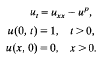Hostname: page-component-7dc689bd49-6fmns Total loading time: 0 Render date: 2023-03-20T21:13:43.933Z Has data issue: true Feature Flags: { "useRatesEcommerce": false } hasContentIssue true

# Short time behaviour of a singular solution to the heat equation with absorption

Published online by Cambridge University Press:  14 November 2011

## Synopsis

The paper considers the small time evolution of the interface which appears in the boundary value problemA diffusion dominated small time outer expansion is not uniformly valid when x = O(t½ log t) and has to be supplemented by an inner expansion valid in a region where diffusion and absorption are of equal importance. For p < 1 a zero order inner solution is constructed, which is consistent with a moving interface where u is zero, and the small time development of this is given.

These results are first derived in a heuristic way using the method of matched expansions. However, an important aim of the paper is to give rigorous proofs, being one of the very few cases in nonlinear diffusion where this has been done.

Type
Research Article

## Access options

Get access to the full version of this content by using one of the access options below. (Log in options will check for institutional or personal access. Content may require purchase if you do not have access.)

## References

1Aris, R.. The mathematical theory of diffusion and reaction in permeable catalysts (Oxford: Clarendon Press, 1975).Google Scholar
2Aronson, D. G., Crandall, M. G. and Peletier, L. A.. Stabilization of solutions of a degenerate nonlinear diffusion problem. Nonlinear Anal. 6 (1982), 10011022.CrossRefGoogle Scholar
3Bandle, C. and Stakgold, I.. Reaction-diffusion and dead cores. In Free boundary problems: applications and theory IV, eds. Bossavit, A., Damlamian, A. and Fremond, M., pp. 436448 (London: Pitman, 1985).Google Scholar
4Bandle, C. and Stakgold, I.. The formation of the dead core in parabolic reaction-diffusion equations. Trans. Amer. Math. Soc. 286 (1984) 275293.CrossRefGoogle Scholar
5Diaz, J. I. and Hernandez, J.. Some results in the existence of free boundaries for parabolic reaction-diffusion systems (Preprint).Google Scholar
6Friedman, A.. Partial differential equations of parabolic type (Englewood Cliffs: Prentice-Hall, 1964).Google Scholar
7Grundy, R. E.. Asymptotic solutions of a model nonlinear diffusion equation. IMA J. Appl. Math. 31 (1983), 121137.CrossRefGoogle Scholar
8Kalashnikov, A. S.. The propagation of disturbances of nonlinear heat conduction with absorption. USSR Comput. Math, and Math. Phys. 14 (1974), 7085.CrossRefGoogle Scholar
9Kamin, S. (Kamenomostkaya, S.). The asymptotic behaviour of the solution of the filtration equation. Israel J. Math. 14 (1973), 7687.Google Scholar
10Kamin, S. and Peletier, L. A.. Source-type solutions of degenerate diffusion equations with absorption. Israeli. Math. 50 (1985), 219230.CrossRefGoogle Scholar
11Kamin, S. and Rosenau, P.. Propagation of thermal waves in an inhomogeneous medium. Comm. Pure Appl. Math. 34 (1981), 831852.CrossRefGoogle Scholar
12Ladyzenskaya, O. A., Solonnikov, V. A. and Uralt'ceva, N. N.. Linear and quasilinear equations of parabolic type. AMS Translations of Mathematical Monographs 23 (1968).CrossRefGoogle Scholar
13Nayfeh, A. H.. Perturbation methods. (New York: J. Wiley, 1973).Google Scholar
14Okubo, A.. Diffusion and ecological problems: Mathematical models (Berlin: Springer, 1980).Google Scholar
15Peletier, L. A. and Tesei, A.. Diffusion in homogeneous media: localization and positivity. Ann. Mat. Pura Appl. (4) 141 (1985), 219229.CrossRefGoogle Scholar
16Protter, M. H. and Weinberger, H. F.. Maximum principles in differential equations (Englewood Cliffs: Prentice-Hall, 1967).Google Scholar
17Dyke, M. van. Perturbation methods in fluid mechanics (New York: Academic Press, 1964).Google Scholar